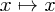You are currently only viewing entries from July 2012.

To go back to view all items, click here. Also, see August 2012 and June 2012
Monday, 16 July 2012

## Temporarily Redefining In-built Mathematica Functions

A neat trick # Permalink C Comment

Suppose a package you're using is, say, zealously Simplifying lots of Mathematica expressions inefficiently, and you'd rather do that later yourself. Can you prevent this from happening?

Yes, very easily, by using the Block environment, so long as you don't need access to the Simplify function in your new, temporary definition:

`NiceFunction[arg_] := Block[{Simplify = Function[x, x]}, NastyFunction[arg]]`

This code redeclares Simplify as being the 'pure function'and then evaluates NastyFunction. A simple example to test this:

`NastyFunction[arg_] := Simplify[arg + Sin[h]^2]NastyFunction[Cos[h]^2] (* outputs 1 *)NiceFunction[Cos[h]^2]  (* outputs Cos[h]^2+Sin[h]^2 *)Simplify[%]             (* outputs 1 *)`

What about if you need to be able to use the original function?

## More Sophisticated Needs

If you still want access to the old definition of the method you're modifying in your new version of the function, you could do something like this:

`ClearAll[DoItNicely];SetAttributes[DoItNicely, HoldFirst];DoItNicely[code_] := Module[{\$inNicely},    Internal`InheritedBlock[{Simplify},        Unprotect[Simplify];        Simplify[arg_] :=             Block[{\$inNicely = True},                 Print["I can still Simplify: " <> ToString[Simplify[arg]]];                arg            ] /; ! TrueQ[\$inNicely];        Protect[Simplify];        code    ]];`

We use that (originating from a useful answer I found after reading an excellent Stack Overflow thread on Mathematica tricks) like the following:

`NastyFunction[arg_] := Simplify[arg + Sin[h]^2]NastyFunction[Cos[h]^2]DoItNicely[NastyFunction[Cos[h]^2]] Simplify[%]`

which produces the output

`1I can still Simplify: 1Cos[h]^2 + Sin[h]^21`

This uses a variable \$inNicely (which must not be used elsewhere!) to determine whether to use the original definition or the new one, and the undocumented Internal`InheritedBlock to completely copy the original function into the new context so that it can be locally modified. To change this for your own purposes, just replace Simplify with the name of the function you're interested in, and modify the two lines of code Print[...]; arg to what you want the new function to do. (You might need to change the number of arguments to the function too, which is fine. Remember that to match arbitrary numbers of arguments use a double underscore after the variable name, args__.).

Posted by carl at 11:38

Filed under: Computing